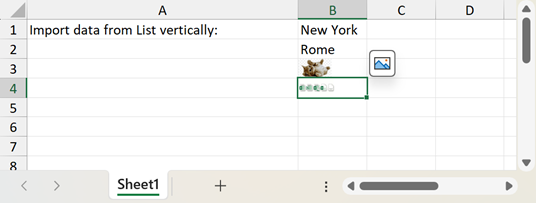The requested page is not available for the requested platform. You are viewing the content for .NET Framework 4.5.2+ platform.

## Mobile

19.2
19.1
18.2
18.1
17.2
.NET Framework 4.5.2+
.NET Framework 4.5.2+
.NET Standard 2.0+
.NET Core 3.0+

# WorksheetExtensions.Import(Worksheet, IEnumerable, Int32, Int32, Boolean) Method

Imports data from a collection.

You require a license to the DevExpress Office File API or DevExpress Universal Subscription to use this method in production code.

Assembly: DevExpress.Docs.v19.2.dll

## Declaration

``````public static void Import(
this Worksheet sheet,
IEnumerable source,
int firstRowIndex,
int firstColumnIndex,
bool isVertical
)``````
``````<ExtensionAttribute>
Public Shared Sub Import(
sheet As Worksheet,
source As IEnumerable,
firstRowIndex As Integer,
firstColumnIndex As Integer,
isVertical As Boolean
)``````
``````public static void Import(
this Worksheet sheet,
IEnumerable source,
int firstRowIndex,
int firstColumnIndex,
bool isVertical
)``````
``````<ExtensionAttribute>
Public Shared Sub Import(
sheet As Worksheet,
source As IEnumerable,
firstRowIndex As Integer,
firstColumnIndex As Integer,
isVertical As Boolean
)``````
``````public static void Import(
this Worksheet sheet,
IEnumerable source,
int firstRowIndex,
int firstColumnIndex,
bool isVertical
)``````
``````<ExtensionAttribute>
Public Shared Sub Import(
sheet As Worksheet,
source As IEnumerable,
firstRowIndex As Integer,
firstColumnIndex As Integer,
isVertical As Boolean
)``````

## Parameters

Name Type Description
sheet Worksheet

A Worksheet that is the worksheet to which the data is imported.

source IEnumerable

An object that exposes the IEnumerable interface provided by a collection of objects being imported.

firstRowIndex Int32

An integer that is the row index of the start cell in which the imported data will be inserted.

firstColumnIndex Int32

An integer that is the column index of the start cell in which the imported data will be inserted.

isVertical Boolean

true, to insert imported data vertically; otherwise, false

## Parameters

Name Type Description
sheet Worksheet

A Worksheet that is the worksheet to which the data is imported.

source IEnumerable

An object that exposes the IEnumerable interface provided by a collection of objects being imported.

firstRowIndex Int32

An integer that is the row index of the start cell in which the imported data will be inserted.

firstColumnIndex Int32

An integer that is the column index of the start cell in which the imported data will be inserted.

isVertical Boolean

true, to insert imported data vertically; otherwise, false

## Parameters

Name Type Description
sheet Worksheet

A Worksheet that is the worksheet to which the data is imported.

source IEnumerable

An object that exposes the IEnumerable interface provided by a collection of objects being imported.

firstRowIndex Int32

An integer that is the row index of the start cell in which the imported data will be inserted.

firstColumnIndex Int32

An integer that is the column index of the start cell in which the imported data will be inserted.

isVertical Boolean

true, to insert imported data vertically; otherwise, false

## Remarks

The following code snippet illustrates how to use the Import method.

``````' Create a List object containing string values.
Dim cities As New List(Of String)()